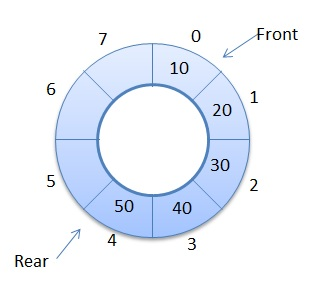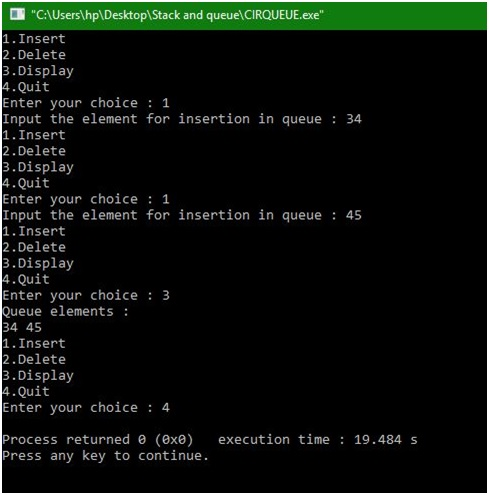# Circular queue using array

In this article, we are going to learn how to implement a circular queue by using array in data structure?
Submitted by Manu Jemini, on December 21, 2017

A circular queue is a very important data structure because it can store data in a very practical way. The circular queue is a linear data structure. It follows FIFO principle. In circular queue, the last node is connected back to the first node to make a circle. Circular array list fallows the First In First Out principle. Elements are added at the rear end and the elements are deleted at the front end of the queue.Image source: - http://scanftree.com/Data_Structure/circularqueues.png

In the Code below there are four parts. First three function to implement three different operations like Insert a node, delete a node and display the list. The Fourth part is the main function, in that a do while loop is implemented to keep the user engaged and provide him the all the given choices, according to the choice one of the three function get called.

The First function checks whether the queue is empty, rear is at last position of queue or Queue is full. If the queue is not full it adds up the item.

The Second function checks whether the list is empty, full or it has only one item. If any node exists, it will delete the node in FIFO order.

The Third function will simply print all the elements of the Queue if exist. If not, then it will say Queue is Empty.

The Queue can hold only 5 items, for changing the capacity edit the second line.

## C program to implement circular queue using array

```# include<stdio.h>
# define MAX 5

int cqueue_arr[MAX];
int front = -1;
int rear = -1;

/*Begin of insert*/
void insert(int item)
{
if((front == 0 && rear == MAX-1) || (front == rear+1))
{
printf("Queue Overflow \n");
return;
}
if (front == -1)  /*If queue is empty */
{
front = 0;
rear = 0;
}
else
{
if(rear == MAX-1)	/*rear is at last position of queue */
rear = 0;
else
rear = rear+1;
}
cqueue_arr[rear] = item ;
}
/*End of insert*/

/*Begin of del*/
void del()
{
if (front == -1)
{
printf("Queue Underflow\n");
return ;
}
printf("Element deleted from queue is : %d\n",cqueue_arr[front]);
if(front == rear) /* queue has only one element */
{
front = -1;
rear=-1;
}
else
{
if(front == MAX-1)
front = 0;
else
front = front+1;
}
}
/*End of del() */

/*Begin of display*/
void display()
{
int front_pos = front,rear_pos = rear;
if(front == -1)
{
printf("Queue is empty\n");
return;
}
printf("Queue elements :\n");
if( front_pos <= rear_pos )
while(front_pos <= rear_pos)
{
printf("%d ",cqueue_arr[front_pos]);
front_pos++;
}
else
{
while(front_pos <= MAX-1)
{
printf("%d ",cqueue_arr[front_pos]);
front_pos++;
}
front_pos = 0;
while(front_pos <= rear_pos)
{
printf("%d ",cqueue_arr[front_pos]);
front_pos++;
}
}
printf("\n");
}
/*End of display*/

/*Begin of main*/
int main()
{
int choice,item;
do
{
printf("1.Insert\n");
printf("2.Delete\n");
printf("3.Display\n");
printf("4.Quit\n");

scanf("%d",&choice);

switch(choice)
{
case 1 :
printf("Input the element for insertion in queue : ");
scanf("%d", &item);

insert(item);
break;
case 2 :
del();
break;
case 3:
display();
break;
case 4:
break;
default:
printf("Wrong choice\n");
}
}while(choice!=4);

return 0;
}
/*End of main*/
```

Output